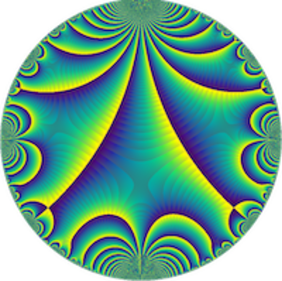# How can one specify a number field? (Jean-Marc Couveignes, Université de Bordeaux)

Séminaire « Arithmétique »
M2 Salle de réunion
There are various ways to specify a number field $\mathbf K$ but no canonical one in general.
A natural possibility is to provide a multiplication table for a $\mathbf \ZZ$-basis of $\mathcal O$, the ring
of integers of $\mathbf K$. Another most standard possibility is to provide the minimal polynomial $F(X)$ of some integer $\alpha$ that is a primitive element of $\mathbf K$ over $\mathbf Q$. These are more or less redundant representations. There is nothing like a convenient complete set of invariant for number fields, and even less canonical models in general. Experimental data suggest that the number of isomorphism classes of number fields with fixed degree $n$ and discriminant bounded by $H$ grows linearly in $H$. This has been proved for $n=3$ by Davenport and Heilbronn and for $n=4, 5$ by Bhargava. I will present a few tools introduced by various authors to specify and count number fields efficiently.